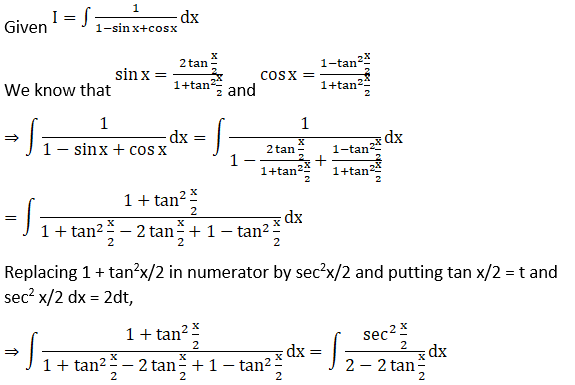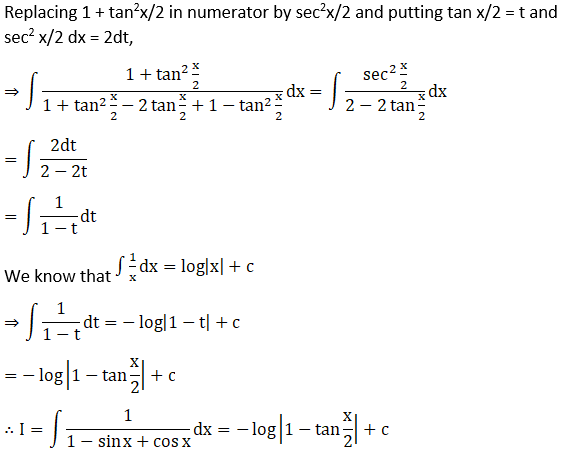# RD Sharma Solutions For Class 12 Maths Exercise 19.23 Chapter 19 Indefinite Integrals

RD Sharma Solutions for Class 12 Maths Exercise 19.23 Chapter 19 Indefinite Integrals is provided here. Determining the integral of the form

$$\begin{array}{l}\int \frac{1}{\sqrt{a \sin x+b \cos x}}\ dx, \int \frac{1}{a+b \sin x}\ dx\end{array}$$
is the main topic dealt with in this exercise. It is very important for the students to have strong knowledge about the concepts covered in each chapter of Class 12, as it’s a turning point in a student’s life.

To make the process easier for students, BYJU’S has created the RD Sharma Solutions for Class 12 Maths, which is a valuable resource for students. After all, it’s advantageous to obtain a good score in a subject like Mathematics. The RD Sharma Solutions are made available in PDF format, so that the students can download it.

## Download the PDF of RD Sharma Solutions For Class 12 Chapter 19 – Indefinite Integrals Exercise 19.23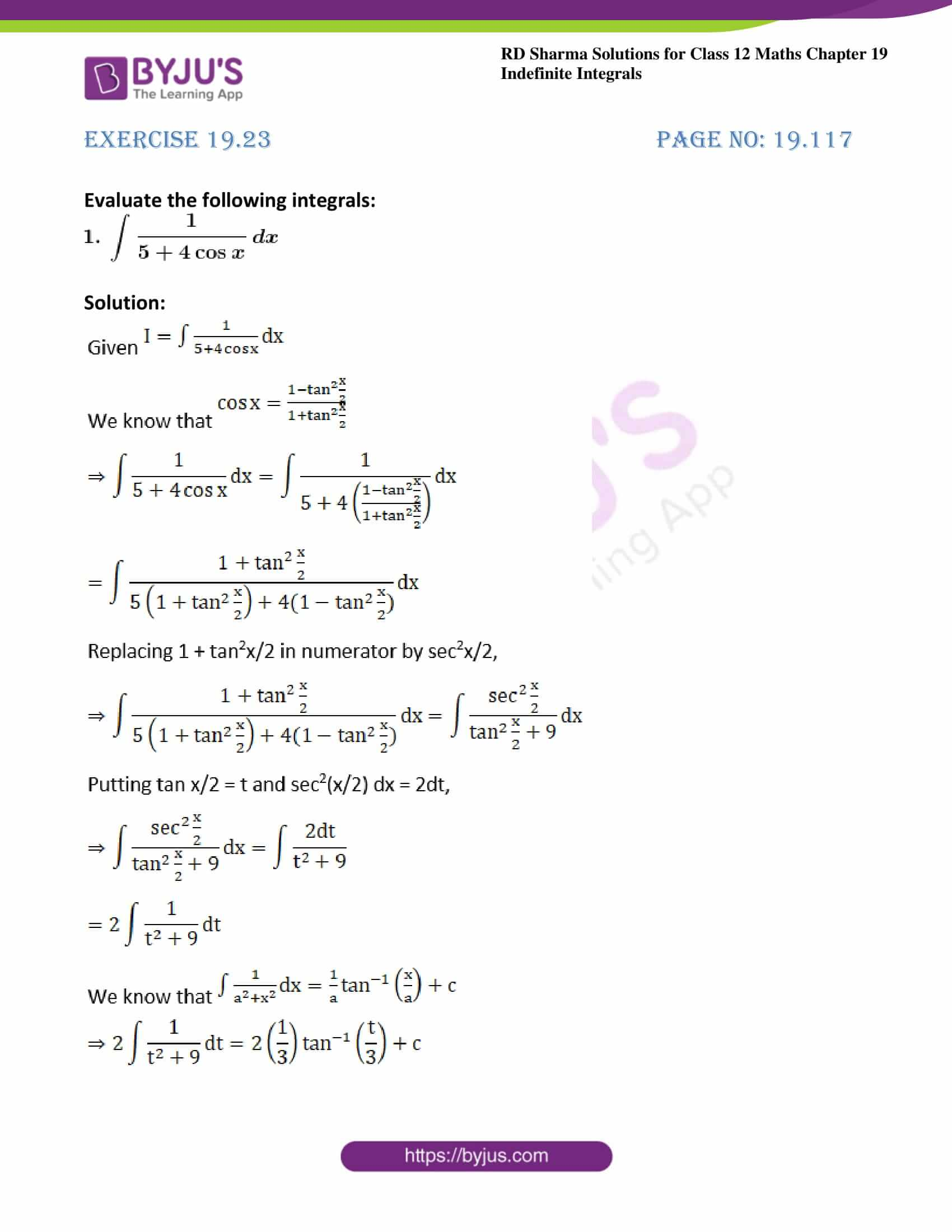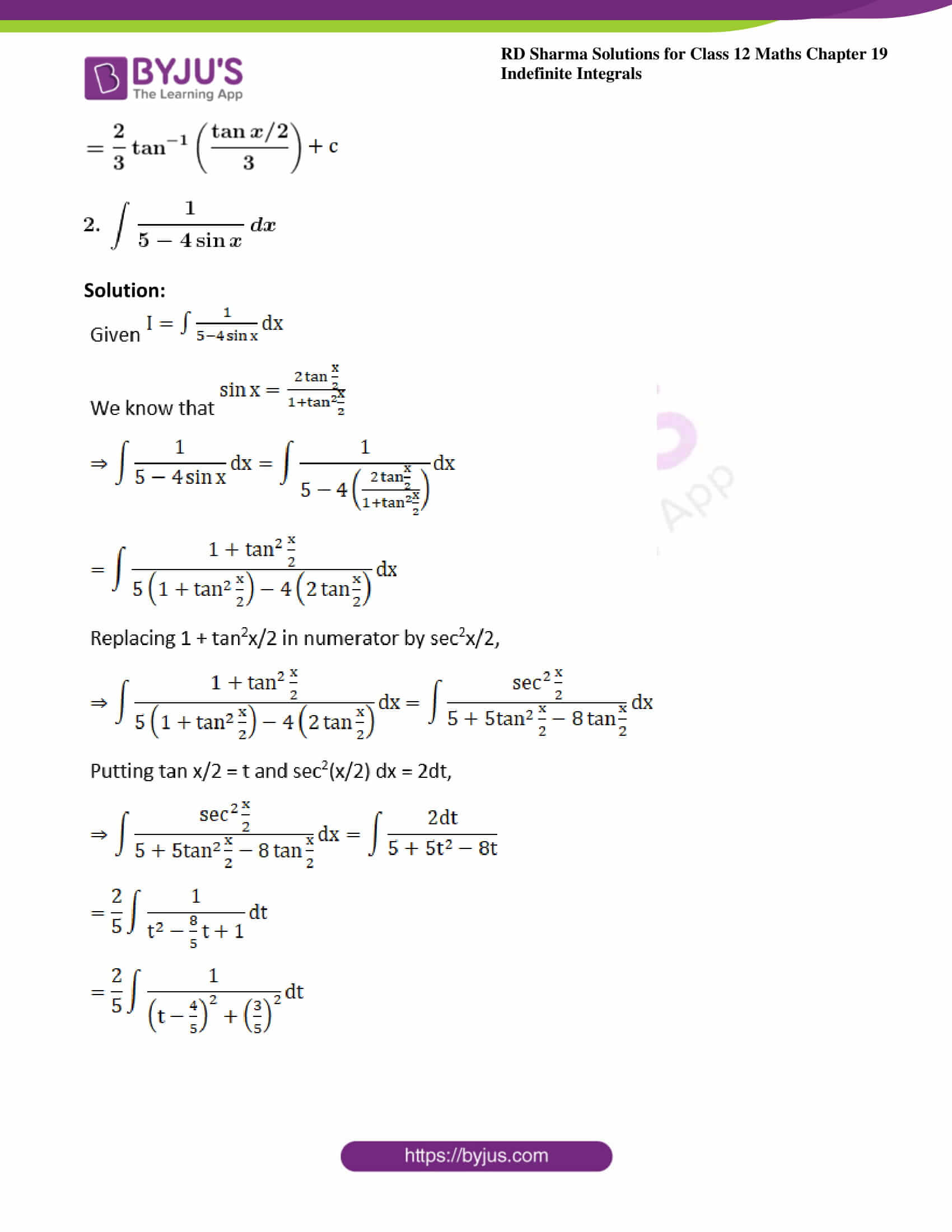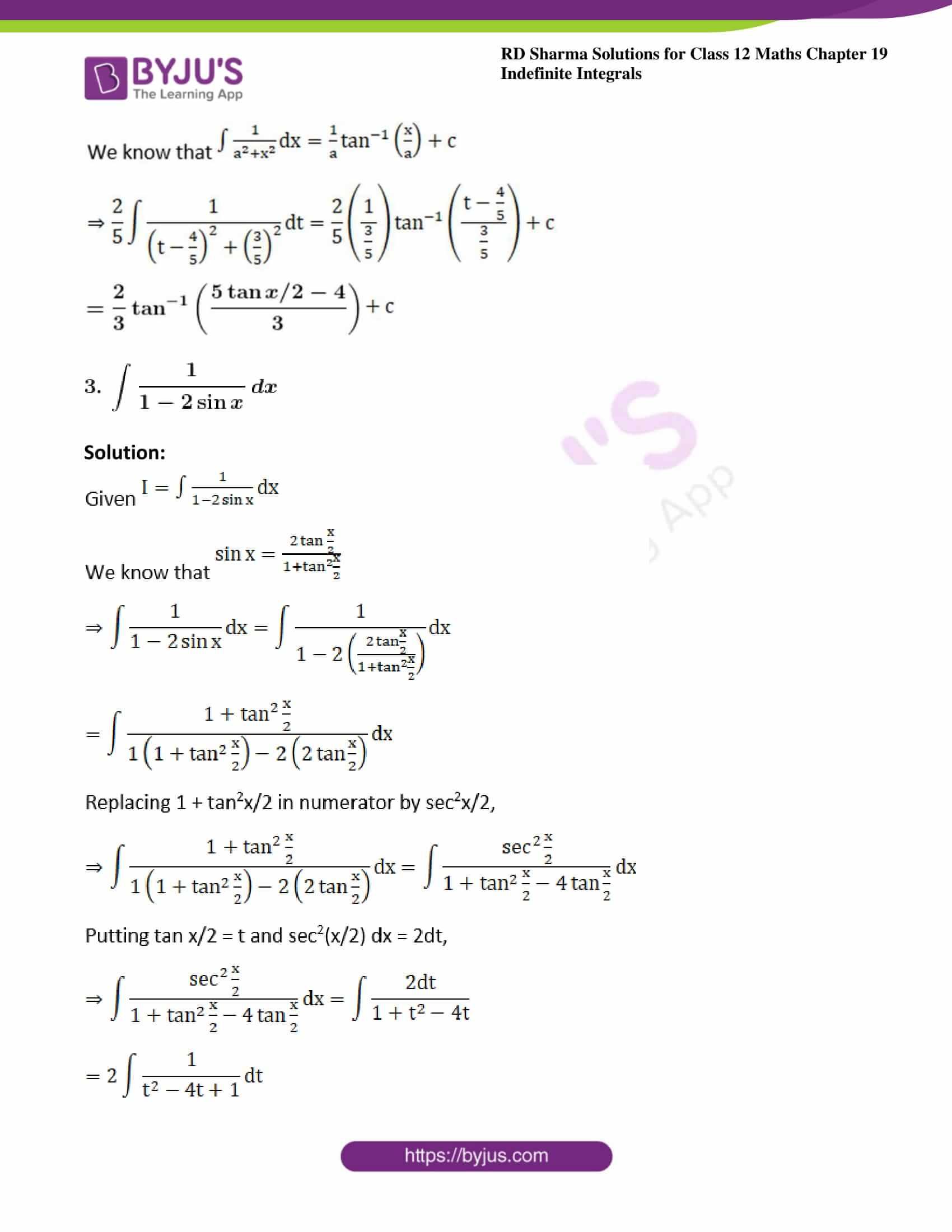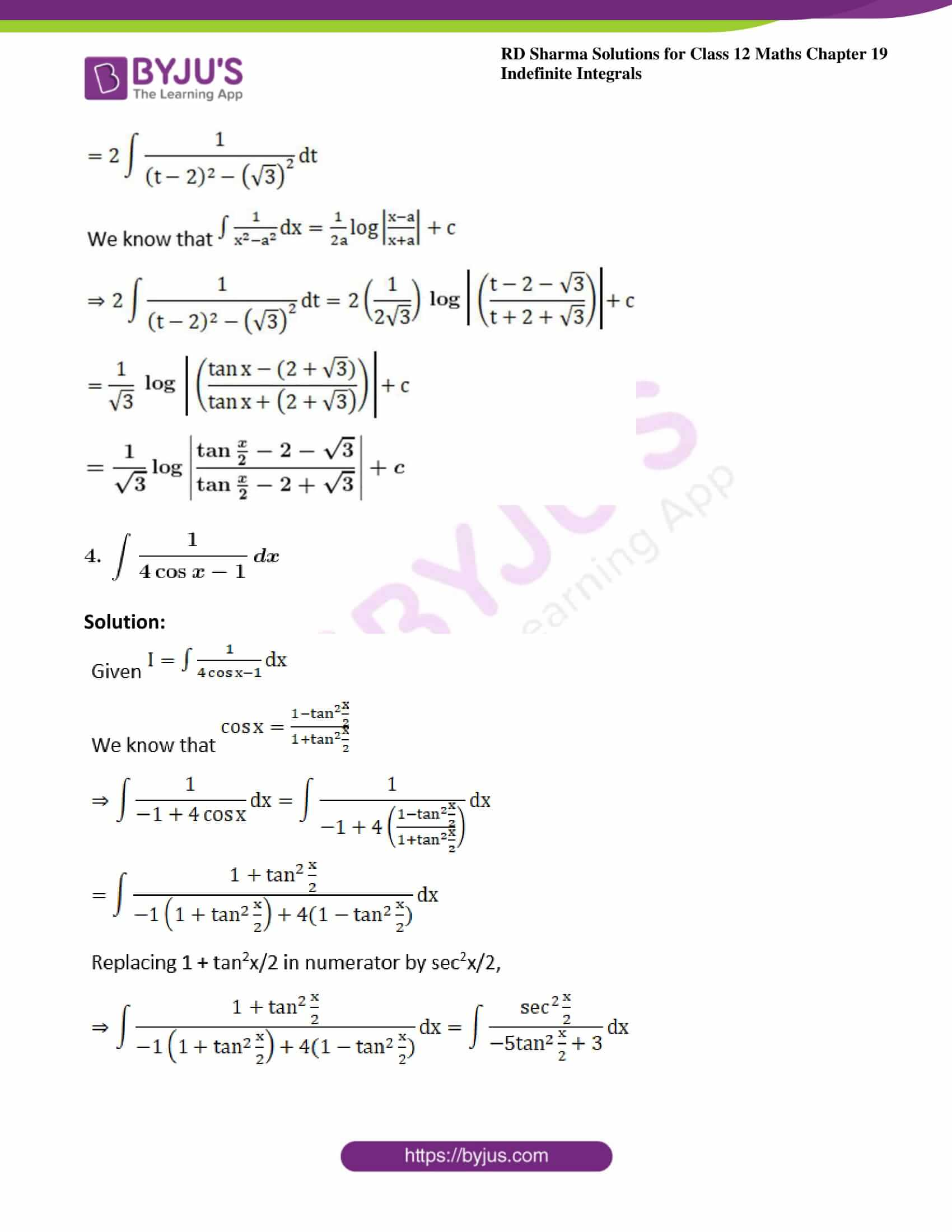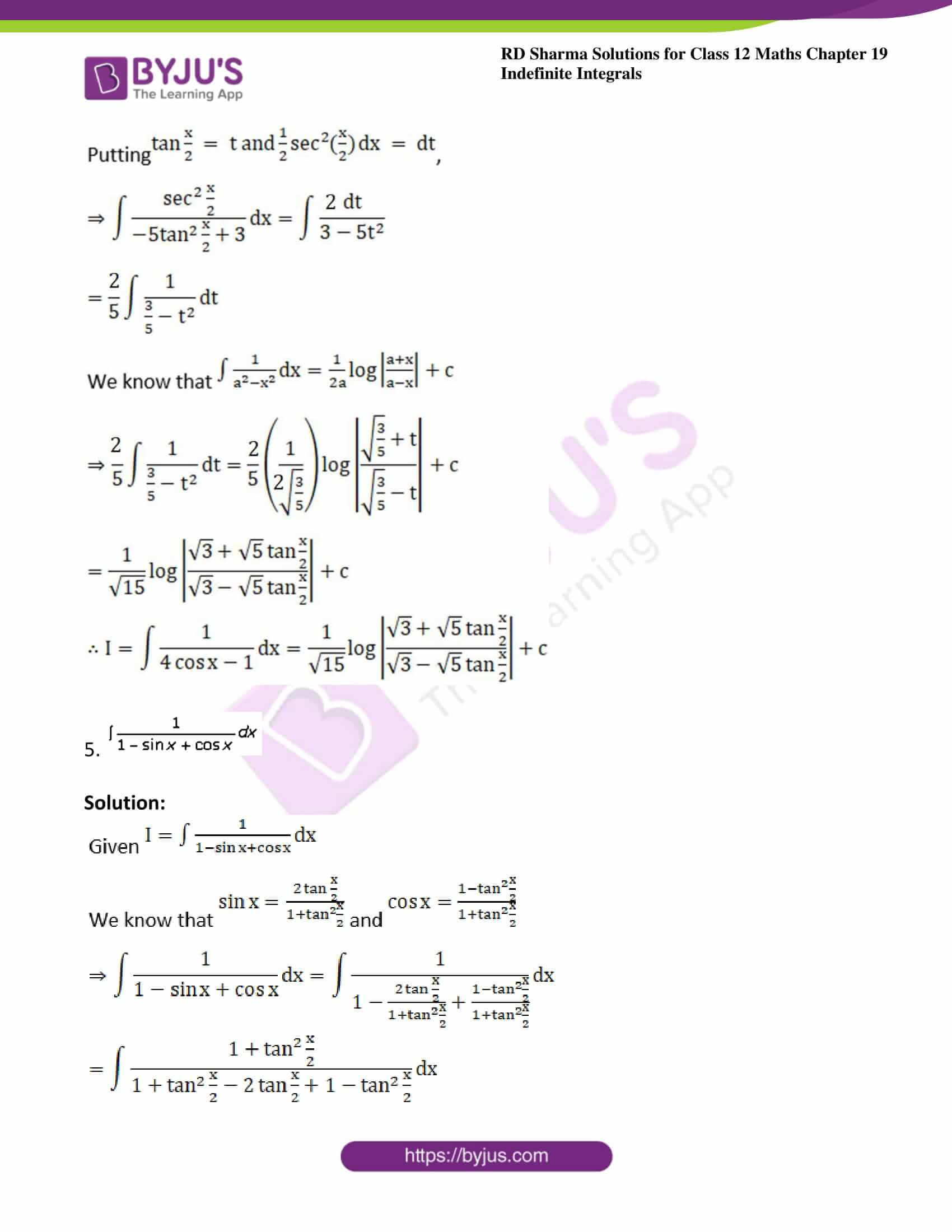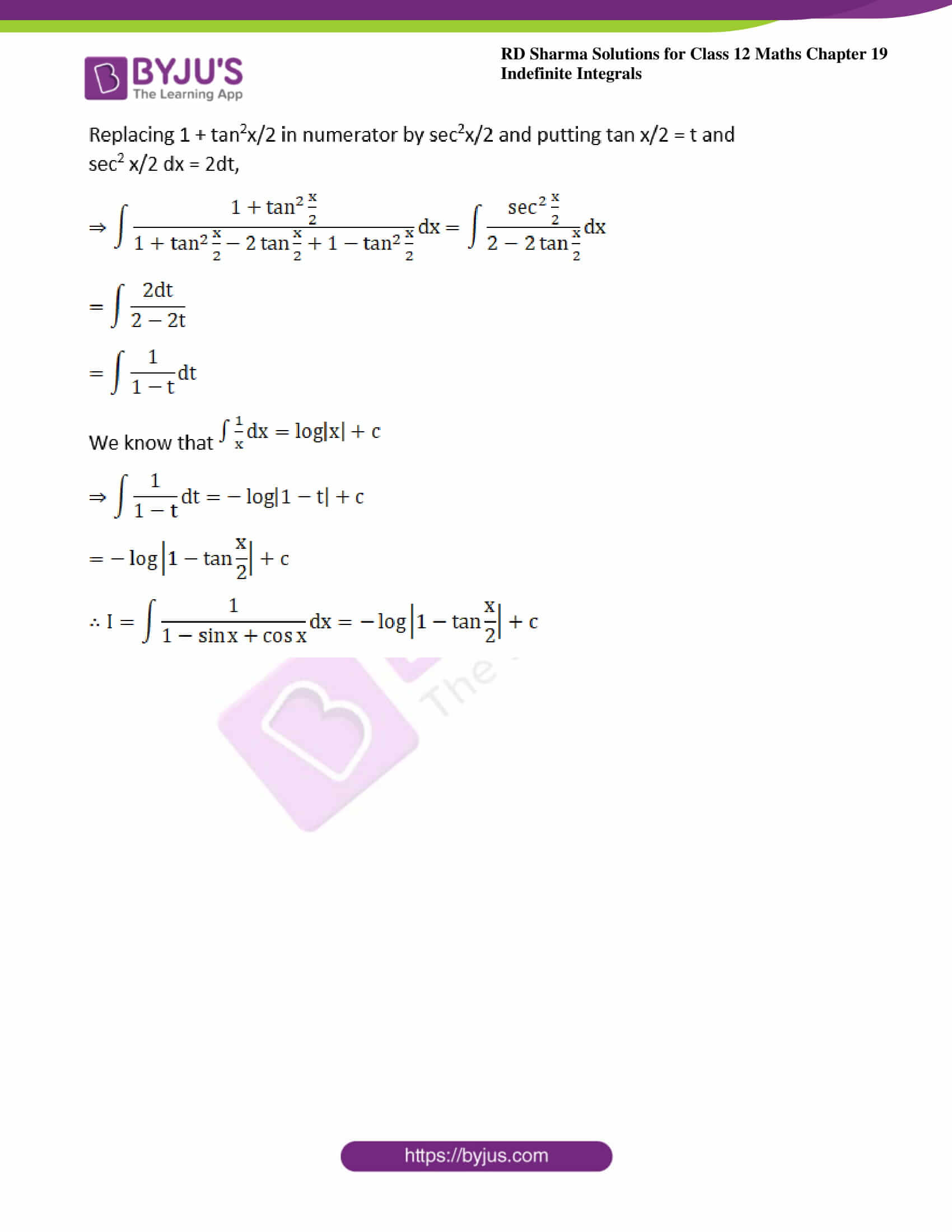### Access other exercises of RD Sharma Solutions For Class 12 Chapter 19 – Indefinite Integrals

Exercise 19.1 Solutions

Exercise 19.2 Solutions

Exercise 19.3 Solutions

Exercise 19.4 Solutions

Exercise 19.5 Solutions

Exercise 19.6 Solutions

Exercise 19.7 Solutions

Exercise 19.8 Solutions

Exercise 19.9 Solutions

Exercise 19.10 Solutions

Exercise 19.11 Solutions

Exercise 19.12 Solutions

Exercise 19.13 Solutions

Exercise 19.14 Solutions

Exercise 19.15 Solutions

Exercise 19.16 Solutions

Exercise 19.17 Solutions

Exercise 19.18 Solutions

Exercise 19.19 Solutions

Exercise 19.20 Solutions

Exercise 19.21 Solutions

Exercise 19.22 Solutions

Exercise 19.24 Solutions

Exercise 19.25 Solutions

Exercise 19.26 Solutions

Exercise 19.27 Solutions

Exercise 19.28 Solutions

Exercise 19.29 Solutions

Exercise 19.30 Solutions

Exercise 19.31 Solutions

Exercise 19.32 Solutions

### Exercise 19.23 Page No: 19.117

Evaluate the following integrals: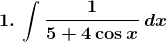Solution: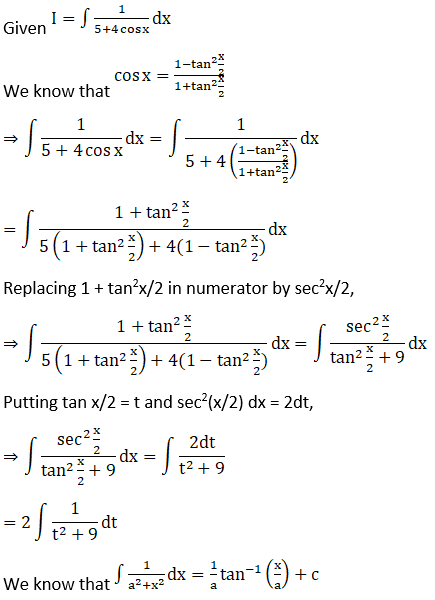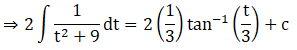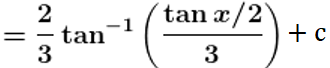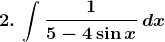Solution: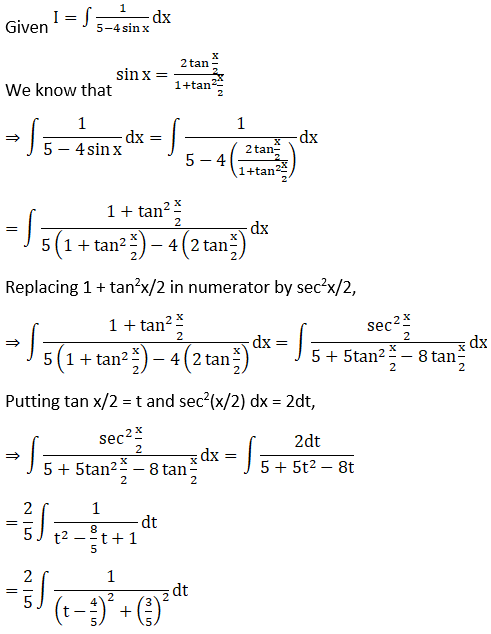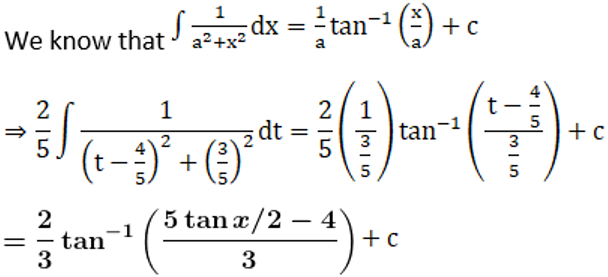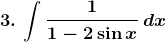Solution: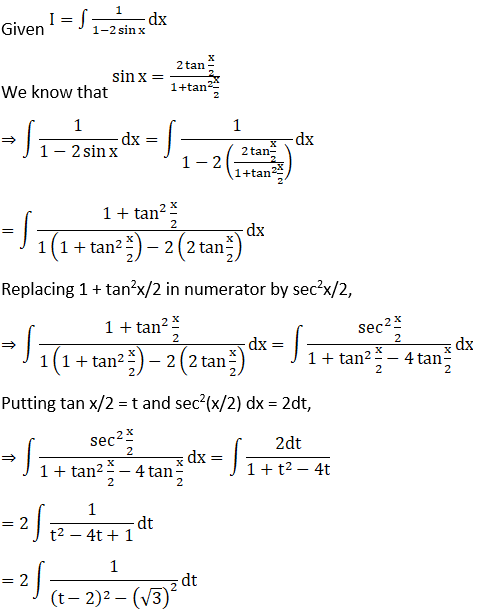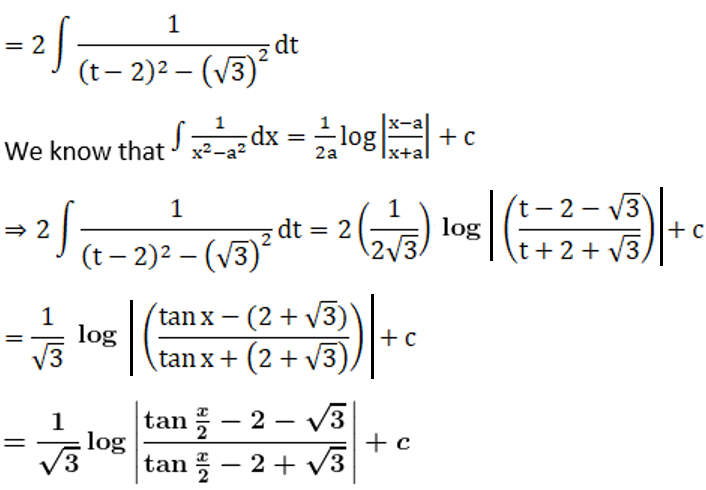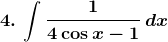Solution: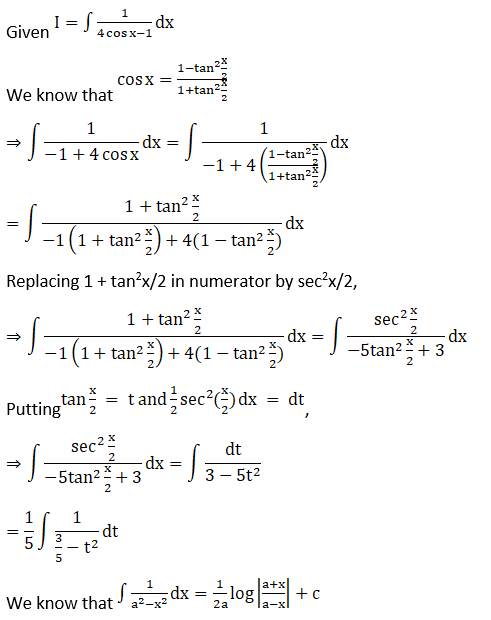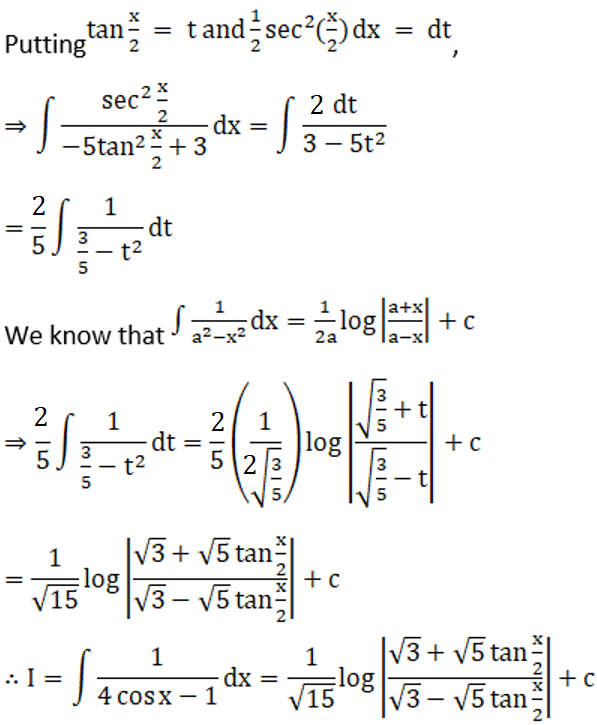5.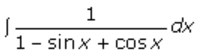Solution: